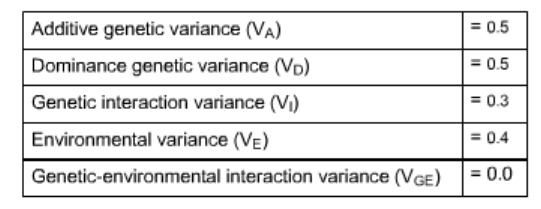# Problem: Suppose that phenotypic variation in the tail length of mice is summarized in the table below.What is the narrow-sense heritability (h2) of tail length? What is the broad-sense heritability (H2) of tail length?

###### FREE Expert Solution

Broad Sense Heritability: estimates percentage of genetic variance using all components of genetic variance

: ratio of genotypic variance to phenotypic variance

Narrow Sense Heritability: estimates percentage of genetic variance using only additive variance

: ratio of additive genotypic variance to phenotypic variance

Variance Components of a Quantitative Trait:

VP = VG + VE + VGE

where: VP = phenotypic variance
VG = genetic variation
VE = environmental variation
VGE = variation associated with the genetic and environmental factor interactions###### Problem Details

Suppose that phenotypic variation in the tail length of mice is summarized in the table below.What is the narrow-sense heritability (h2) of tail length?

What is the broad-sense heritability (H2) of tail length?

Frequently Asked Questions

What scientific concept do you need to know in order to solve this problem?

Our tutors have indicated that to solve this problem you will need to apply the Heritability concept. You can view video lessons to learn Heritability. Or if you need more Heritability practice, you can also practice Heritability practice problems.